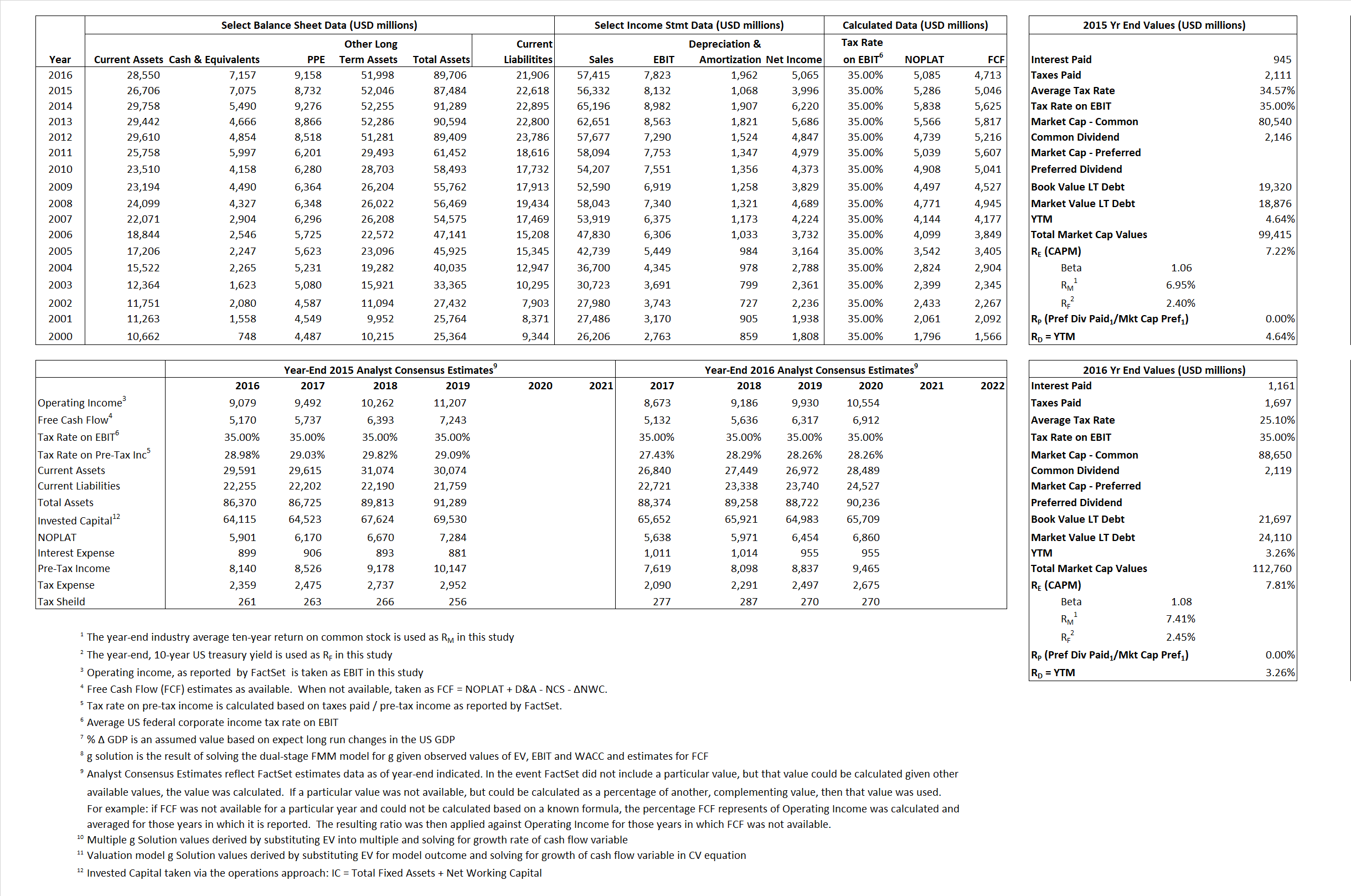# United Technologies Corporation

## Analyst Listing

The following analysts provide coverage for the subject firm as of May 2016:

 Broker Analyst Analyst Email Morningstar Barbara Noverini barbara.noverini@morningstar.com Langenberg, LLC Brian K. Langenberg brian@langenberg-llc.com Cowen & Company Cai von Rumohr cai.von-rumohr@cowen.com Bernstein Research Douglas S. Harned douglas.harned@bernstein.com Jefferies Howard A. Rubel hrubel@jefferies.com Argus Research John Eade jeade@argusresearch.com Credit Suisse Julian Mitchell julian.mitchell@credit-suisse.com Deutsche Bank Research Myles Walton myles.walton@db.com William Blair Nicholas P. Heymann nheymann@williamblair.com CRT Capital Group Peter Arment parment@sterneageecrt.com Atlantic Equities Richard Radbourne r.radbourne@atlantic-equities.com Stifel Nicolaus Robert P. McCarthy mccarthyr@stifel.com RBC Capital Markets Robert Stallard robert.stallard@rbccm.com Wells Fargo Securities Sam J. Pearlstein sam.pearlstein@wellsfargo.com

## Primary Input Data## Derived Input Data

### Equational Form

Net Operating Profit Less Adjusted Taxes NOPLAT 5,286 5,085$NOPLAT\, =\, EBIT\, x\, (1 \,-\, Avg \,\,Tax\,\, Rate\,\, on\,\, EBIT)$
Free Cash Flow FCF 5,046 4,713$FCF\,=NOPLAT\,+\,Non-Cash\,Expenses-\Delta NWC\,-\,NCS$
Tax Shield TS 327 291$TS\,=\,Interest\,\,Paid\,\,x\,\, Avg \,\,Tax\,\,Rate\,\, on\,\, Pre-Tax\,\, Income$
Invested Capital IC 64,866 67,800$IC\,=\,Fixed\,\,Operating\,\,Assets\,\,+\,\,Net\,\, Working\,\, Capital$
Return on Invested Capital ROIC 8.15% 7.50%$ROIC\,=\,\frac { NOPLAT }{ IC }$
Net Investment NetInv (2,460) 4,896$NetInv\,=\,{ {IC}_{1}}-{{IC}_{0}}+Depreciation$
Investment Rate IR -46.54% 96.28%$IR\,=\,\frac {NetInv}{NOPLAT}$
Weighted Average Cost of Capital
WACCMarket 6.43% 6.66%$WACC\,=\,\frac { E }{ V } { R }_{ E }\,+\,\frac { P }{ V } { R }_{ P }\,+\,\frac { D }{ V } { R }_{ D }\left( 1- Avg\,\, Tax\,\,Rate\,\,on\,\,Pre-Tax\,\,Income \right)$
WACCBook  8.26%  7.99%
Enterprise value
EVMarket 92,340 105,603$EV\,=\,Market\,\,Cap\,\,Equity\,+\,\,Long\,\,Term\,\,Debt\,-\,Cash$
EVBook  92,703  103,190
Long-Run Growth
g = IR x ROIC
-3.79% 7.22% Long-run growth rates of the income variable are used in the Continuing Value portion of the valuation models.
g = %$\Delta$ GDP  2.50%  2.50%
Margin from Operations M 14.44% 13.63%$M\,\,=\,\,\frac{EBIT}{SALES}$
Depreciation/Amortization Rate D 11.61% 20.05%$D\,\,=\,\,\frac{D+A}{EBITDA}$

## Valuation Multiple Outcomes

The outcomes presented in this study are the result of original input data, derived data, and synthesized inputs.

### model g solution

12/31/2015 12/31/2016 12/31/2015 12/31/2016 12/31/2015 12/31/2016

EV/SALES$\frac {EV}{Sales} \,= \,\frac{ROIC\, -\, g}{ROIC\,(WACC\,-\,g)}\,(1\,-\,T)\,(M)$

1.64  1.84  2.37%  5.15%  2.27%  2.27%

EV/EBITDA$\frac {EV}{EBITDA} \,= \,\frac{ROIC\, -\, g}{ROIC\,(WACC\,-\,g)}\,(1\,-\,T)\,(1\,-\,D)$

10.04 10.79 2.37% 5.15% 2.27% 2.27%

EV/NOPLAT$\frac {EV}{NOPLAT} \,= \,\frac{ROIC\, -\, g}{ROIC\,(WACC\,-\,g)}$

17.47 20.77 2.37% 5.15% 2.27% 2.27%

EV/FCFOPS$\frac {EV}{FCF_{OPS}} \,= \,\frac{ROIC\, -\, g}{ROIC\,(WACC\,-\,g)}\,(1\,-\,T)$

18.30 22.41 2.37% 5.15% 2.27% 2.27%

EV/EBIT$\frac {EV}{EBIT} \,= \,\frac{ROIC\, -\, g}{ROIC\,(WACC\,-\,g)}\,(1\,-\,T)$

11.36 13.50 2.37% 5.15% 2.27% 2.27%

EV/IC$\frac {EV}{IC} \,= \,\frac{ROIC\, -\, g}{WACC\,-\,g}$

1.42 1.56 2.37% 5.15% 2.27% 2.27%